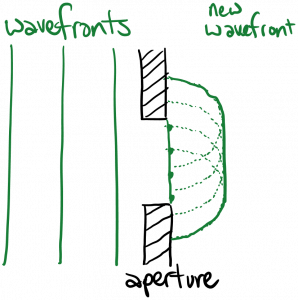Laser Photonics Directionality and diffraction

# Directionality and diffraction

In the last section, I told you that the plane waves we’ve written were perfectly monochromatic, because they each had a single frequency.  It’s also true that the plane waves we’ve written are perfectly unidirectional, because they each have a single direction of propagation.  And just as a purely monochromatic wave is unphysical because it extends from in time from$-\infty$ to$\infty$, it is also true that a purely unidirectional wave is unphysical, because it extends in space from$-\infty$ to$\infty$.

I also said it is possible to construct a more physically realistic, finite duration wave by summing up (or integrating over) single-frequency plane waves.  Similarly, it is possible to construct a more physically realistic, finite-sized beam by summing up (or integrating over) a set of plane waves with different directions of propagation.  (This is the subject of Fourier Optics, the math of which is beyond our scope at the moment.  But we can discuss some of the general principles.)  Since a single plane wave has infinite wavefront size, constructing a beam with a finite-sized waist will require adding several plane waves.  The smaller the waist size, the more plane waves we’ll have to add in.    But if we add in more plane waves propagating in different directions, this means that the beam is less unidirectional, and therefore the beam will spread out more as it propagates.  This is a fundamental property of light waves, called diffraction:  the more we ‘squeeze’ a beam, the more the beam will ‘spread’ as it travels away.

Another way of modeling diffraction is Huygen’s principle.  This principle states that every point on a wavefront acts like the source of a spherical wave moving forward.  We can sum up all these spherical waves to find the next wavefront.  If the starting wavefront is perfectly planar and infinite in size, the next wavefront will be planar as well:However, if we constrict the size of the wavefront by passing the wave through an aperture, subsequent wavefronts will be curved on the edges:Thus, passing a wave through an aperture, as shown in the second figure above, will cause the beam to spread as it moves forward.  Mathematically, Huygen’s principle can be expressed as$\displaystyle E(x, y, z = d) = C \int \int _{- \infty}^{\infty} E(x', y', z = 0) \frac{e^{ik \sqrt{(x-x')^2 + (y - y')^2 + d^2}}}{\sqrt{(x-x')^2 + (y-y')^2 + d^2}} dx' dy'$

In this expression, we’re basically convolving the electric field at$z = 0$ with a spherical wave.  The spherical wave is acting like the impulse response function of the system, where in this case the system is light propagation in free space.  This equation is tricky to use in practice, so luckily we have a much simpler approximation to fall back on.  If we define$\Phi$ as the angle of divergence of a beam, as shown in the diagram below:Then for beams with very small divergence (such as most laser beams) we can approximately write$\displaystyle \sin{\Phi} = 2.44 \frac{\lambda}{D}$

where$D$ is the diameter of the aperture that the beam is passing through, or the diameter of the beam in some plane.  Waves measure the size of physical features relative to their own wavelength.  Notice that the denominator of this equation contains$D / \lambda$, which is the ratio of the aperture diameter to the wavelength.  As that ratio gets smaller, the divergence angle increases (diffraction increases).

Laser beams tend to be highly directional – they exhibit very little divergence.  This is due to the way in which laser light is produced (stimulated emission) as well as the geometry of most laser cavities, as we’ll discuss later.

Here’s a lovely video demonstrating diffraction with water waves:

https://www.youtube.com/watch?v=BH0NfVUTWG4

Aside:

You might remember that ‘squeezing’ a time-domain function has the effect of ‘spreading’ its frequency-domain Fourier transform, which is mathematically similar to the diffraction we’re describing.  This is also the basis of Heisenberg’s famous Uncertainty Principle.